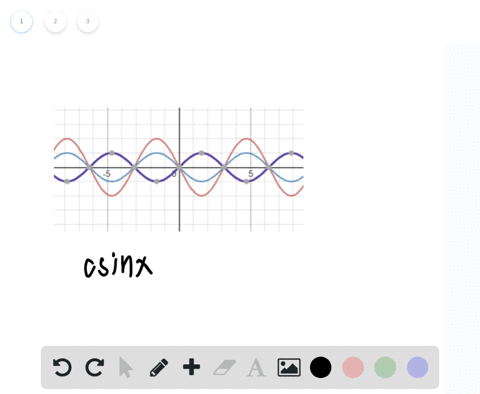🎉 The Study-to-Win Winning Ticket number has been announced! Go to your Tickets dashboard to see if you won! 🎉View Winning Ticket### Writing In Exercises 53 and $54,$ use a graphing …

00:21University of Southern California
Problem 52

# Period In Exercises $49-52,$ find the period of the function.$$y=\csc 4 x$$

## Discussion

You must be signed in to discuss.

## Video Transcript

the problem asks us to find the period of the following function. Why equals coast? He cant of four x Now when we want to calculate the period of the coast, he can function. All we have to do is use the following formula Period equals to pie over the absolute value of being Where bee in this instance is that term that's adjacent to X are independent variable inside of our trick in a metric function. So since in this case, B equals four, we simply have to write to pi divided by four equals R period and upon simplification and equals pi over too. So the period of Kosi can four x is pie.